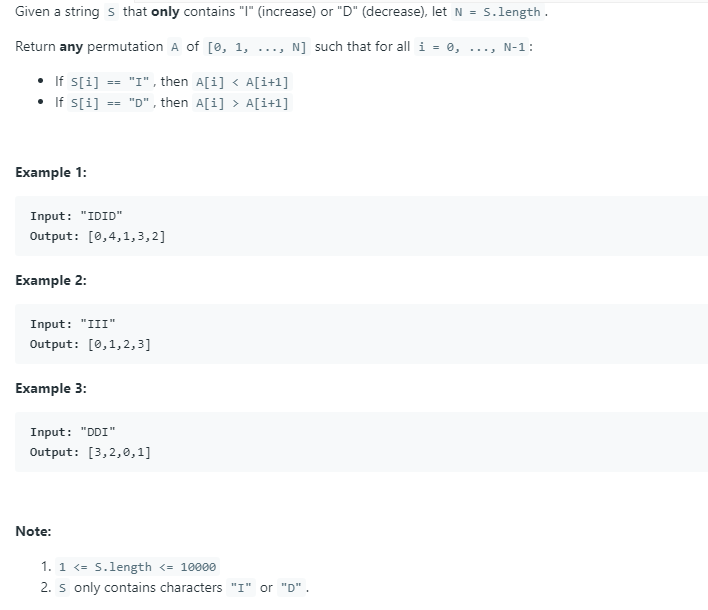## 2012年1月31日 星期二

### [Perl 文章收集] 如何在 perl 子函數中傳遞 hash

1. too expensive. 整個 hash 是被拷貝到函數中, 因此就算你在函數中對該 hash 進行操作, 原本的 hash 並不受影響, 因此需要將修改後的 hash 回傳.
2. 如果有超過一個參數要傳，而把hash放到最前面，結果在子函數中會合併到第一個hash上面去，如 :
1. %relhash = (
2.     1=>"one",
3.     2=>"two",
4.     3=>"three"
5. );
6.
7. \$test = "test";
8.
9. sub printHash()
10. {
11.     my(\$var1, %hash2) = @_;
12.     print "\t[Test] var1=\$var1\n";
13.     for my \$key (keys %hash2)
14.     {
15.         my \$value = \$hash2{\$key};
16.         print "\t[Test] Hash(\$key) -> \$value\n";
17.     }
18.     return %hash2;
19. }
20.
21. &printHash(\$test, %relhash);

[Test] var1=test
[Test] Hash(1) -> one
[Test] Hash(3) -> three
[Test] Hash(2) -> two

1. ...
2. sub printHash()
3. {
4.     my(%hash2, \$var1) = @_;
5.     ...
6. }
7.
8. &printHash(%relhash, \$test);

[Test] var1=
[Test] Hash(test) ->
[Test] Hash(1) -> one
[Test] Hash(3) -> thr
[Test] Hash(2) -> two

3. 如果同時傳了兩個 hash 或 array，在子函數中也會合併到第一個中去，而第二個就會傳不進來!

1. %relhash = (
2.     1=>"one",
3.     2=>"two",
4.     3=>"three"
5. );
6.
7. \$test = "test";
8.
9. sub printHash()
10. {
11.     my(\$var1, \$hash2) = @_;
12.     print "\t[printHash] var1=\$\$var1\n";
13.     for \$key (keys %\$hash2)
14.     {
15.         \$value = \$hash2->{\$key};
16.         print "\t[printHash] hash2(\$key) -> \$value\n";
17.     }
18.     \$hash2->{4} = "four";
19. }
20.
21. &printHash(\$test, \%relhash); # 傳遞 hash 的 reference
22.
23. while( my(\$k, \$v) = each %relhash)
24. {
25.     print "\t[Main] relhash(\$k) -> \$v\n";
26. }

[printHash] var1=test
[printHash] hash2(1) -> one
[printHash] hash2(3) -> three
[printHash] hash2(2) -> two
[Main] relhash(4) -> four
[Main] relhash(1) -> one
[Main] relhash(3) -> three
[Main] relhash(2) -> two

1. ...
2. processarrays(\@array1, \@array2);
3.
4. \$sub processarrays{
5.    my(\$a1, \$a2) = @_;
6.    foreach(@\$a1){    # dereferences \$a1
7.       print \$_;
8.    }
9.    for(\$i=0; \$i<@\$a2; \$i++){
10.        print \$a2->[\$i];    #get at a particular index within array
11.    }
12. }

Perl 函數傳引用 (Scalar, Array and Hash)

### [LeetCode] Easy - 942. DI String Match

Source From   Here Question How-To view plain copy to clipboard print ? #!/usr/bin/env python3   class   Solution:       def di...